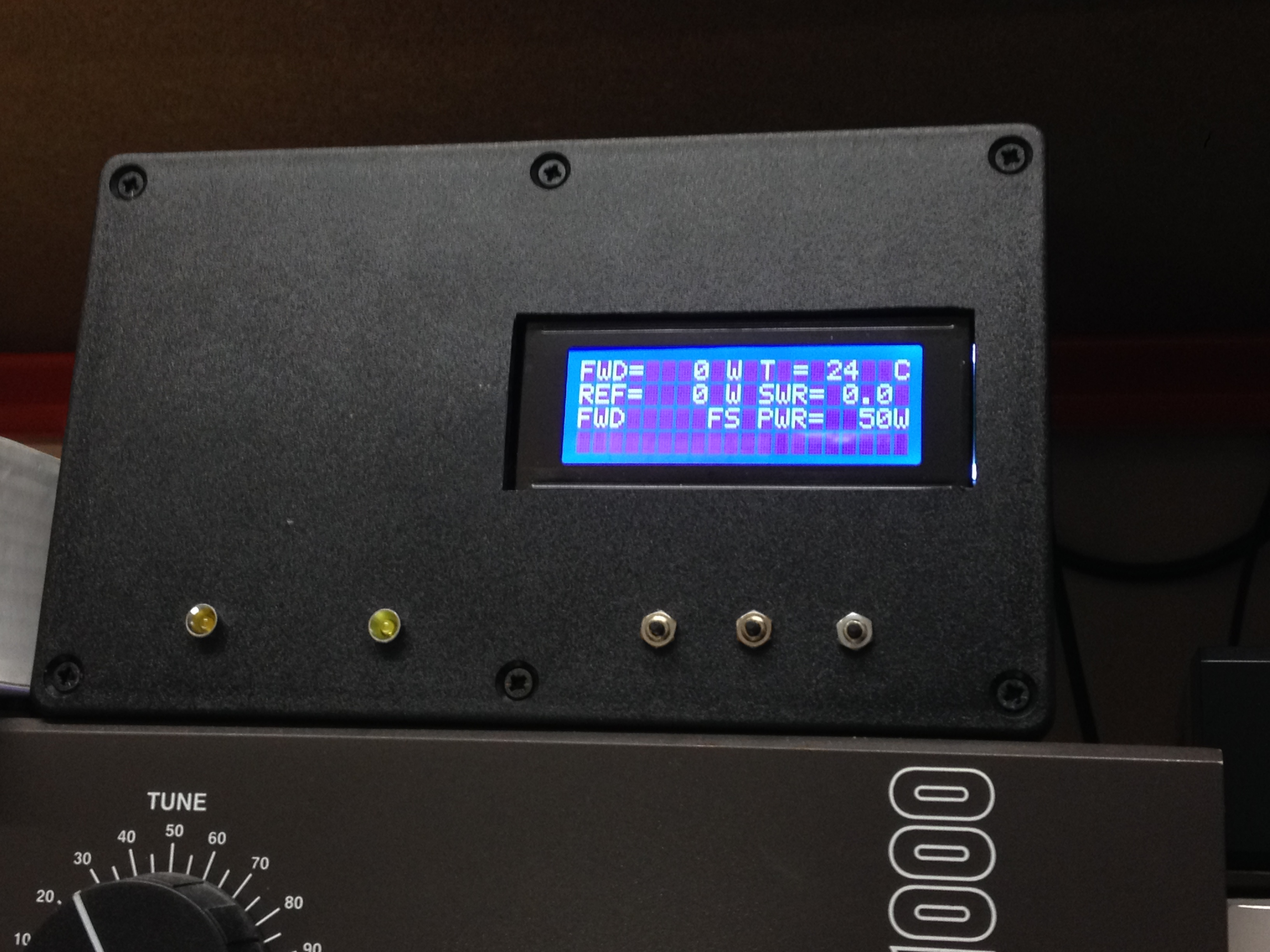# SWR Power meter with protection for Solid State PA

## SWR Power meter with protection for Solid State PA

Also usable as a dual mW meter with a few changes in the software.

#### Introduction

This SWR Power meter was my first own project  for the Arduino. I started it from scratch and decided to build an accurate Power meter for the directional coupler described HERE. This meter had to be suitable for a large power range. I used my experience from early days with the AD8307 log detector chip from Analog Devices. (HERE) This chip is suitable up to 500 MHz and has a very high dynamic range. It can measure almost from almost the noisefloor (-74dBm or even lower)  up to 10 mW where the upper range is less accurate, so we better stay away from that. The measurement accuracy is excellent. With a dynamic range of 88dB the accuracy is 1dB according to the data sheet. This accuracy is also valid for the SWR-Power meter if your calibration is accurate. But even without calibration this power / SWR meter is better than most of the commercial available SWR Power meters.

The first steps showed very nice results and I build a number of SWR-Power meters to replace the older gear in my shack. They worked very well and I am still using them. In a conversation with Wim PA0WMX he suggested a few protection circuit features to be build in as well. More and more the idea developed and it now has SWR protection, temperature protection and a blower speed control. There are also 2 current protection circuits and a voltage protection. This is the maximum I could use with my programming skills, there were no pins left on the Atmega328.

Wim PA0WMX needed some boards and so did I, so we decided to design  a board and we had it made professionally. See some pictures below.The first line of the display shows the forward power and the temperature

The second line shows the reflected power and the calculated SWR

The third line shows the mode and the full scale power of the bar graph which is on the fourth line.

#### Short description of the buttons

The unit has 3 buttons:

#1. is the reset button in case of an alarm, you can only reset the protection circuitry with this button. Alarms are set at SWR 1:2 and temperature at 45 degrees C. You can change these values in the software.  If you push this button it will pause for few seconds and than the greeting screen will show for 1 second.

The greeting screen: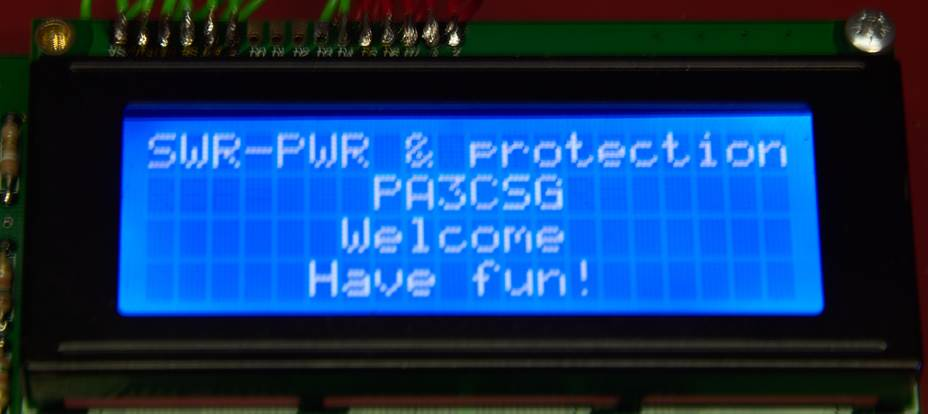#2. Is the Mode button. With this button you will change from Forward ( the third line will show FWD), Second mode is Reflected (REF) and Third mode is Temp. In FWD mode the bargraph will display FWD power, In REF mode the bar graph will display REF power, in third mode the bar graph will show Temperature from 0-100 degrees.

#### The Forward mode

The bar graph displays the forward power. With the “Full Scalle Power” button you can set the full scale power of the bar graph. Mode and full scale power are shown on the third line of the display.#### The Reflected mode

This mode shows the reflected power on the bar graph. Again the full scale power can be set for this mode with the “Full scale power” This allows you to align an antenna very accurate for SWR.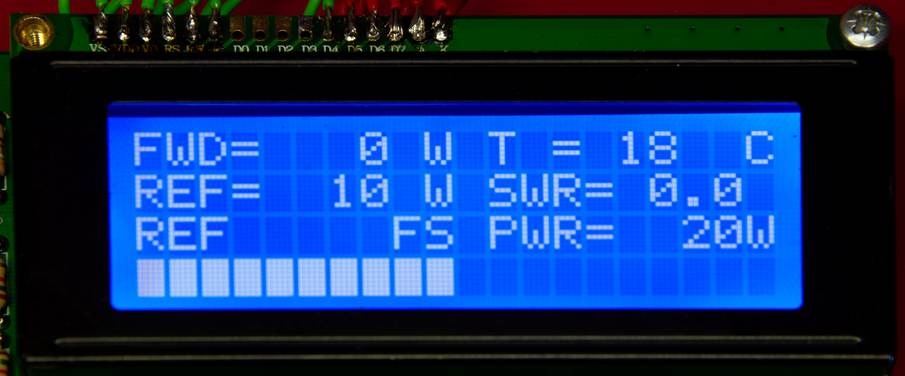#### The temperature mode

Now the bar graph displays the temperature. Note that the full scale temperature is fixed to 100 degrees. In this mode you can watch the temperature rise of your PA.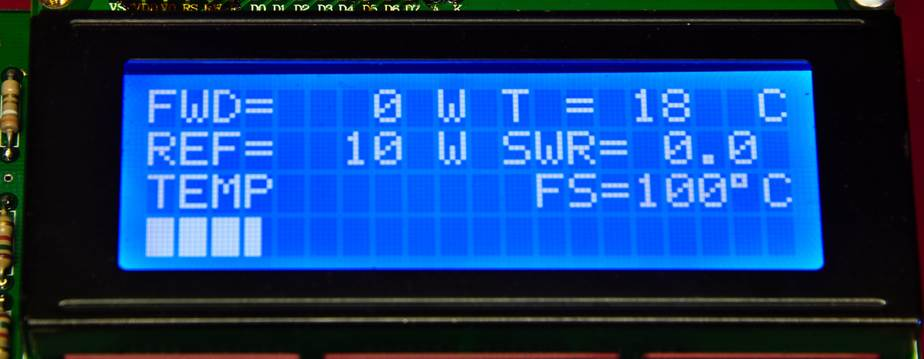#3. This button is the the “full scale power button” It will display the full scale power of the bar graph on the third line to the right. With this button you can scale down (ONLY DOWN) It will automatically scale up when you reach the limit of the bar graph. So when you produce 105W it will go to the 200W full scale position. If you lower your power to say 90W you will have to scale it manually  down to 100W full scale.

The 2 leds display alarm for SWR and temp. They will light in case of high SWR or temperature. The display with show a warning also. This warning will only go away by resetting the PWR SWR meter with the reset button. If for example the temperature has not gone down it will produce immediately another alarm.  If the level  has gone down below the alarm level, it will work again as a protection circuit.

In case of an alarm Pin #3 of theP10 connector will go high as well, you will have to put a 560 Ohm resistor in series with this pin. (It will be on the board for the next version). For now it is not short circuit protected! This alarm pin can switch off your amp or transceiver PTT or whatever. So whenever there is something wrong with your amplifier or your antenna it will automatically switch your station to standby preventing further damage.

The blower control is on Connector P11 pin #3. You need to insert few more components to get a blower running, see schematic. The temperature where the blower will start running and for what percentage is set in the software. This will vary for different blowers. In fact it is a PWM control.

#### Schematic and board

Below is the schematic of the PWR-SWR meter and protection. It is rather small but you can download a PDF AD8307-PWR-meter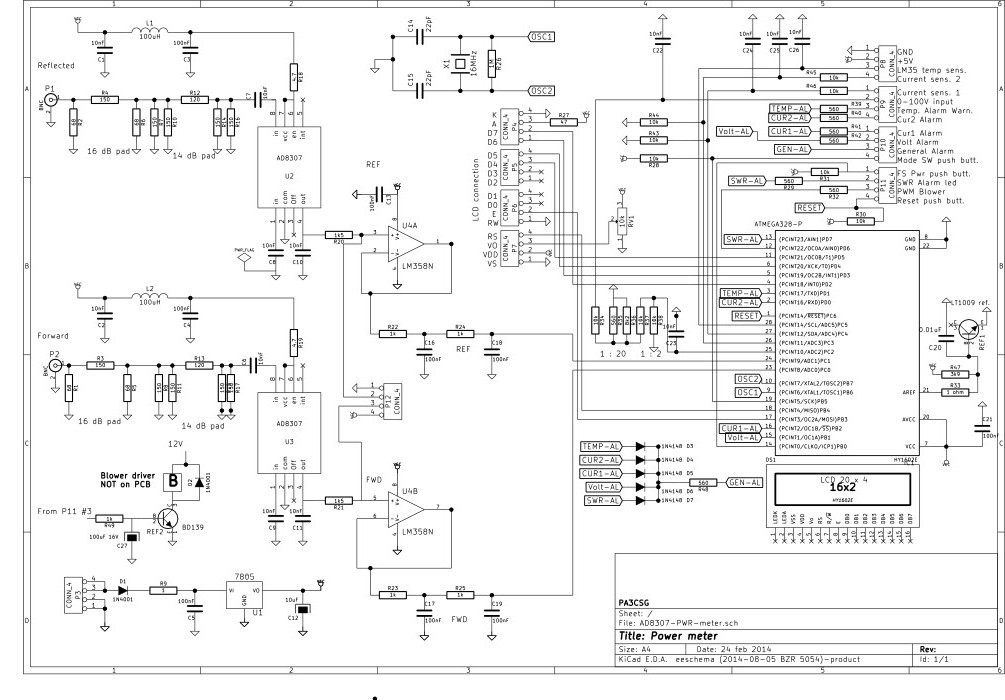In Europe most hams have some amount of grey hair. This is clearly visible on the flea market 🙂

For this reason I decided that the board had to be lumped components. But prices of the AD8307 made me decide different. For a DIL8 \$15 was normal price, for the SMD version it was only \$3 (prices January 2015). The AD8307 in SMD is not very small so with fine solder point and some 0.4mm solder wire it should be possible. Attenuator network was also done in SMD1206 components (except the 2W resistors).Below is a close up of the SMD sectionThe LCD display can be mounted on the top of the board. But you can also make the wires a bit longer and mount it in a different way.

The top view of the board: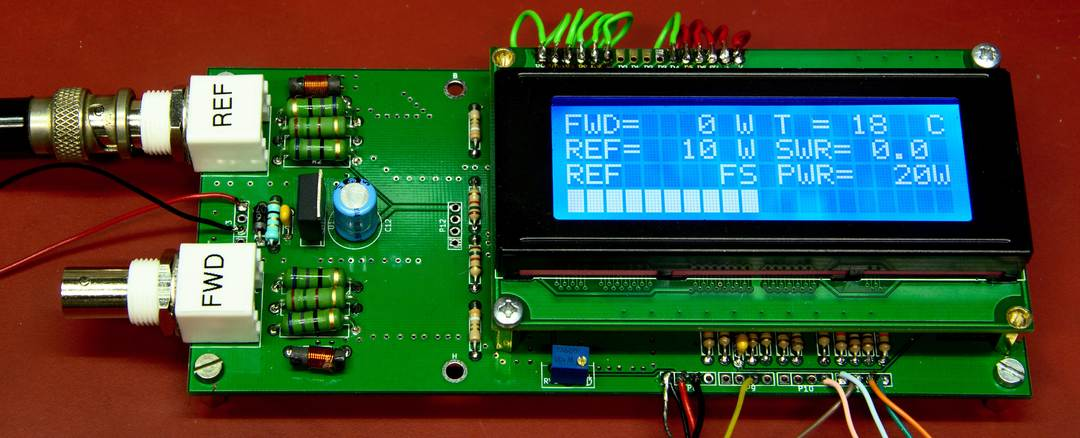Left of the LCD display there are 2 holes. In combination with the left mounting holes a small board with an AD8313 and a small software adaptation the Power SWR meter will be usable up to 2.5 GHz including the 432, 1296 and 2320 MHz frequencies.

The Power SWR meter works very well in combination with the couplers published on the page.

A high power version can be found RF-engineering section, this will work up to 30 MHz

A slightly different version will handle 1kW but will go from 1.5 to 70 MHz. Or from 1.5 to 80 MHz.

There is not much test equipment needed. A DVM should do the job. If you have excess to a signal generator that would make things more accurate.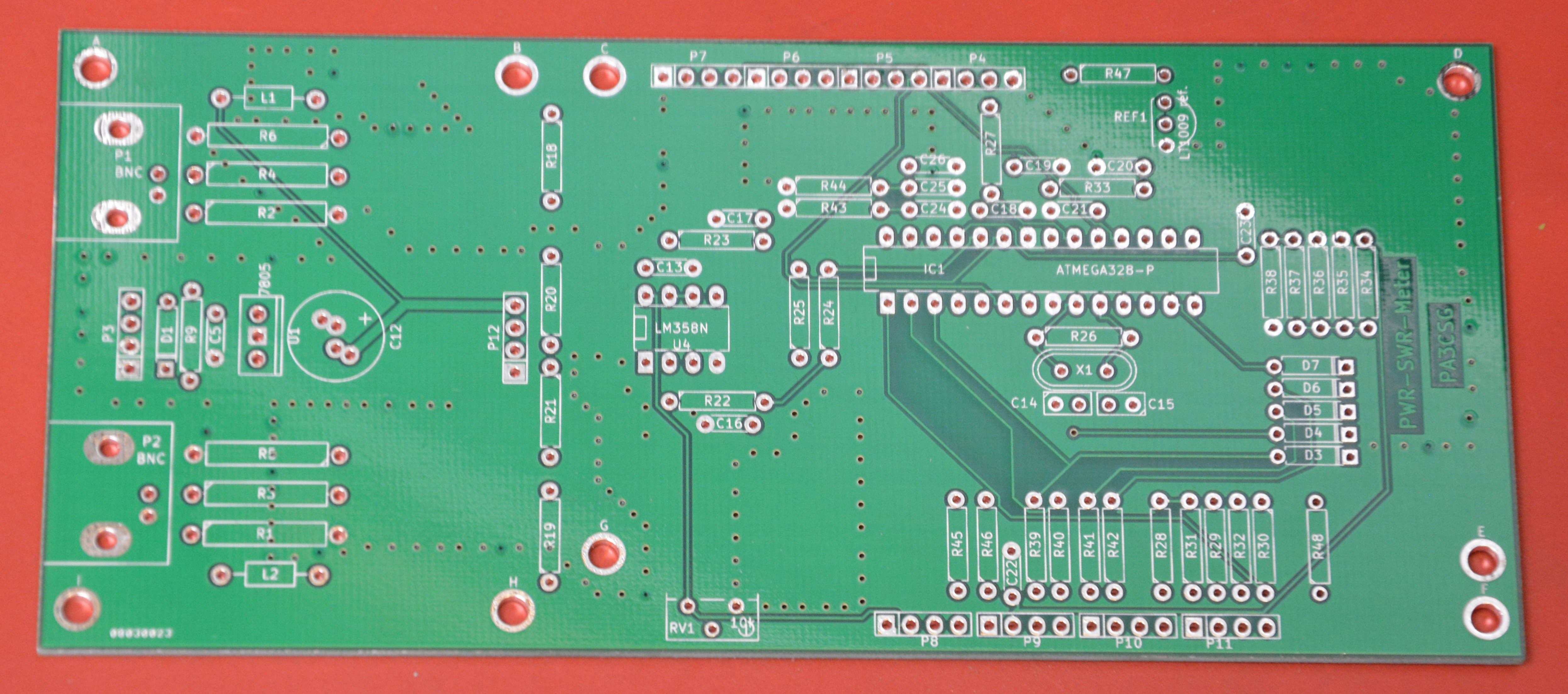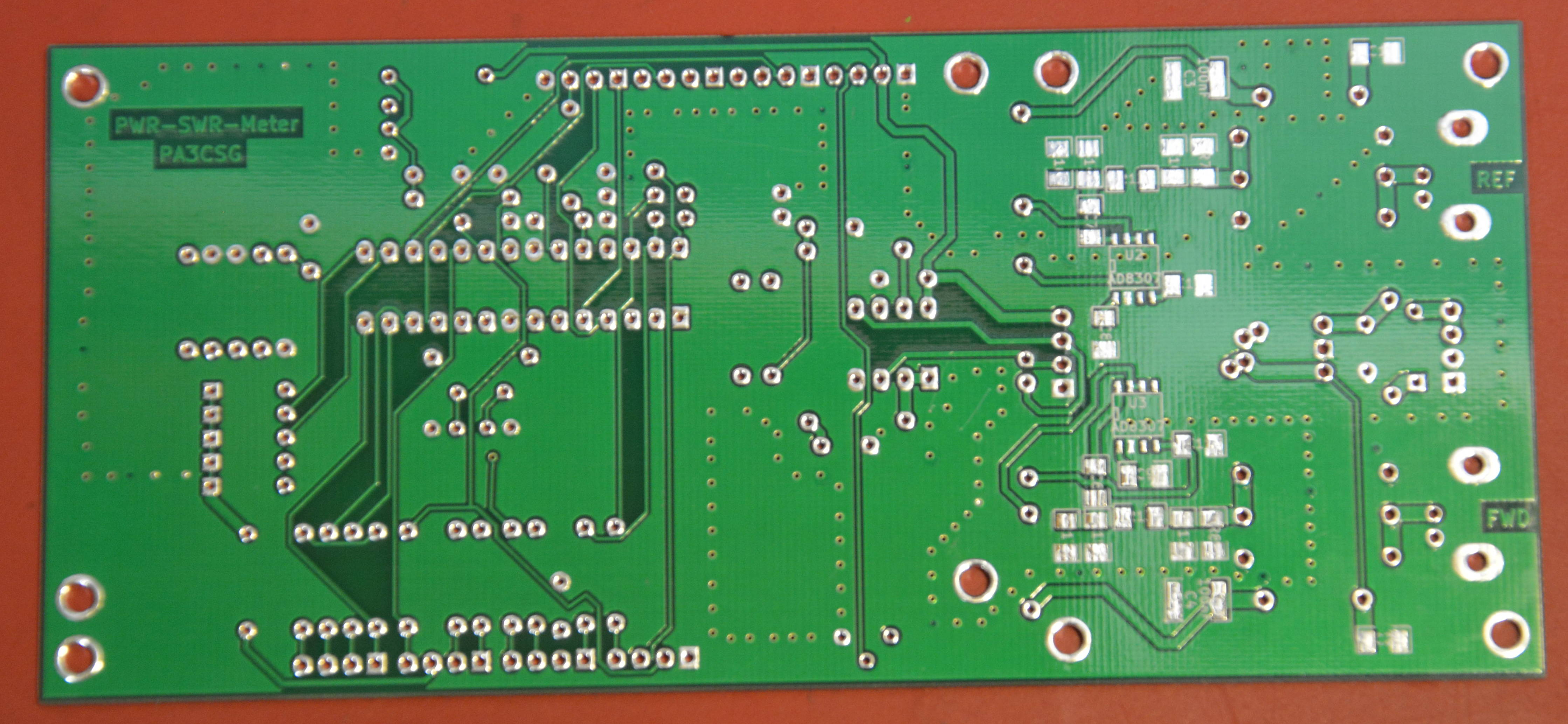Please find the code below! Note that I am not a professional programmer and not even a skilled programmer. If you discover any bugs errors or make improvements please let me know!

` ``/*`
`  `
`  ``Copyright Geert Stams PA3CSG`
` ``All trademarks referred to in source code and documentation are copyright their respective owners.`
`    `
`    ``This program is free software: you can redistribute it and/or modify`
`    ``it under the terms of the GNU General Public License as published by`
`    ``the Free Software Foundation, either version 3 of the License, or`
`    ``(at your option) any later version.`
`    ``This program is distributed in the hope that it will be useful,`
`    ``but WITHOUT ANY WARRANTY; without even the implied warranty of`
`    ``MERCHANTABILITY or FITNESS FOR A PARTICULAR PURPOSE.  See the`
`    ``GNU General Public License for more details.`
`    ``You should have received a copy of the GNU General Public License`
`    ``along with this program.  If not, see <``http:``//www.gnu.org/licenses/>.`
`  `
`  ``************************************************************************`
`  `
`  ``PWR SWR meter & TEMP PA protection + Blower PWM Control`
`  ``PA3CSG January 2015 V1.0`
`  `
`  ``***********************CHANGELOG****************************************`
`  ``14 april 2015 added second measurement you can choose one of two measurements`
`  ``20 april 2015 added low level limits for power, noiselevel of ad8307 might cause swr alarm`
`  ``************************************************************************ `
`*/`
`// include the library code:`
`#include <``LiquidCrystal.h``>`
`#include <``EEPROM.h``>`
`#include <``LcdBarGraph.h``>`
`// initialize the library with the numbers of the interface pins`
`LiquidCrystal lcd(12, 11, 5, 4, 3, 2);`
`// -- creating bargraph instance, format is (&lcd, lcdNumCols, start X, start Y). So (&lcd, 16, 0, 1) would set the bargraph length to 16 columns and start the bargraph at column 0 on row 1.`
`LcdBarGraph lbg(&lcd, 20, 0, 3); `
`// variables for input pin and control LED`
`   ``//variables for the power meter`
`   ``// For the AD converters`
`   `
`  ``int analogInputFWD = 0; //input FWD voltage on pin A0`
`  ``int analogInputREF = 1; //input REF voltage on pin A1`
`  ``int analogInputTemp = 5; //input from LM35 temp sensor`
`  `
`  ``//For the alarms`
`  ``int alarmswr = 7; //SWR alarm on pin 7`
`  ``int alarmtemp = 1; //Temp warning on pin 1`
`  `
`  `
`  ``//For the blower control`
`  ``int fan = 6; // the pin where fan is`
`  ``int tempMin = 25; // the temperature to start the fan`
`  ``int tempMax = 50; // the maximum temperature when fan is at 100%`
`  ``int fanSpeed;`
`  ``int eff;`
`   `
`  ``//float variables`
`  ``float vout2 = 0.0;`
`  ``float vin2 = 0.0;`
`  ``float vout3 = 0.0;`
`  ``float vin3 = 0.0;`
`  ``float out2dbm = 0.0;`
`  ``float out3dbm = 0.0;`
`  ``float RL = 0.0;`
`  ``float coupFWD = 60.4; //coupling factor and attenuators value set before ad8307 mW meter input in dB for FWD`
`  ``float coupREF = 60.4; //coupling factor and attenuators value set before ad8307 mW meter input in dB for REF`
`  ``float wattfwd = 0.0;`
`  ``float wattref = 0.0;`
`  ``float swr = 0.0;`
`  ``float temp = 0.0;`
`  `
`    `
`// variable to store the value `
`  ``int counterPwr = EEPROM.read(0);`
`  ``int counteroldPwr = counterPwr;`
`  ``int counterMode = 0;`
`  ``int counteroldMode = counterMode;`
`  ``int value2 = 0;`
`  ``int value3 = 0;`
`  ``int value2a = 0;`
`  ``int value3a = 0;`
`  ``int updater = 0; `
`  ``int incrementStatePwr = 0; //will read increment for Power button`
`  ``int lastIncrementStatePwr = 0;`
`  ``int incrementStateMode = 0; //will read increment for Mode button`
`  ``int lastIncrementStateMode = 0;`
`  ``byte incrementButtonPwr = 8; // the pin that the pushbutton for the power selection is attached to`
`  ``byte incrementButtonMode = 13; // the pin that the pushbutton for the power selection is attached to`
`        `
`  `
`void setup(){`
`  `
`  ``// declaration of pin modes`
`  ``analogReference(EXTERNAL); //sets reference to external connected reference 2,5V LT1009 reference`
`  `
`  ``// For the AD converters`
`  ``pinMode (analogInputFWD, INPUT); //input from AD8307`
`  ``pinMode (analogInputREF, INPUT); //input from AD8307`
`  ``pinMode (analogInputTemp, INPUT); //input from temp sensor LM35`
`    `
`  `
`  ``//For the pussh buttons`
`  ``pinMode(incrementButtonPwr, INPUT); // initialize the button pin  for the Pwr switch as an input:`
`  ``pinMode(incrementButtonMode, INPUT); // initialize the button pin  for the Mode switch as an input:`
`//for the leds outputs`
`  ``pinMode (alarmswr, OUTPUT); //SWR alarm on pin 7`
`  ``pinMode (alarmtemp, OUTPUT); //Temp warning on pin 1`
`    `
`  ``//setup alarms`
`  ``digitalWrite(alarmswr, LOW);`
`  ``digitalWrite(alarmtemp, LOW);`
`  `
`  `
`  ``//for the PWM control of the blower`
`  ``pinMode(fan, OUTPUT);`
`    `
`  ``// set up the LCD's number of columns and rows:`
`  ``lcd.begin(20, 4);`
`  `
`  ``// A little bit of PR`
`  ``lcd.setCursor(0,0);`
`  ``lcd.print("SWR-PWR & protection");`
`  ``lcd.setCursor(7,1);`
`  ``lcd.print("PA3CSG");`
`  ``lcd.setCursor(6,2);`
`  ``lcd.print("Welcome");`
`  ``lcd.setCursor(5,3);`
`  ``lcd.print("Have fun!");`
`   ``delay(1000);`
`  ``lcd.clear();`
`  `
`  ``//print things on the lcd that donnot change anymore`
`  `
`   ``//first line`
`   ``lcd.setCursor(0, 0);`
`   ``lcd.print("FWD=");`
`   ``lcd.setCursor(9, 0);`
`   ``lcd.print("W");`
`   ``lcd.setCursor(11, 0);`
`   ``lcd.print("T =");`
`   ``lcd.setCursor(18, 0);`
`   ``lcd.print((char)223);`
`   ``lcd.print("C");`
`   `
`    ``//second line`
`   ``lcd.setCursor(0, 1);`
`   ``lcd.print("REF=");`
`   ``lcd.setCursor(9, 1);`
`   ``lcd.print("W");`
`   ``lcd.setCursor(11,1);`
`   ``lcd.print("SWR=");`
`   `
`   `
`   ``//third line is done later`
`   `
`   `
`   ``//fourth line is bargraph`
`} `
`// main calculation routine starts here`
`void loop() {`
` `
`//code for the Power SWR meter  `
`// resets value 2 & 3`
`  `
`    ``value2 = 0;`
`    ``value3 = 0;`
`  `
`  `
`  ``// read the value on analog input 15 times and determine max value`
`      ``for(int i = 0; i <=15; i++) {`
`      ``value2a = analogRead(analogInputFWD);`
`      ``value3a = analogRead(analogInputREF);`
`      ``value2 = max(value2a , value2);`
`      ``value3 = max(value3a , value3);`
`      ``delay(5);`
`    ``} `
`  `
`  ``vout2 = (value2 * 2.5) / 1024.0; //FWD`
`  ``vout3 = (value3 * 2.5) / 1024.0; //REF`
`  `
`  ``//Conversion to DBM the 0.1851 is the output voltage from the AD8307 with -20dbm applied to the input of the chip.`
`  ``//This means that with the 30 dB attenuators on the PCB you have to apply +10dBm at the input BNC connector.`
`  ``//This voltage seems to be more or less the same for most AD8307 if you have no means of measuring leave it like this.`
`  `
`  ``//For the FWD measurement`
`  `
`  `
`   ``if (vout2 >= 1.851) `
`  ``{`
`    ``out2dbm = ((vout2 - 1.851) / 0.025); `
`    ``out2dbm = (-20 + out2dbm);`
`  ``}`
`  `
`    ``if (vout2 < ``1.851``) `
`  ``{`
`    ``out2dbm = ((1.851 - vout2) / 0.025);`
`    ``out2dbm = (-20 - out2dbm);`
`  ``}`
`  `
`  `
`  ``//For the REF measurement`
`  ``if (vout3 < 1.855) `
`  ``{`
`    ``out3dbm = ((1.855 - vout3) / 0.025);`
`    ``out3dbm = (-20 - out3dbm);`
`  ``}`
`  `
`   ``if (vout3 >= 1.855) `
`  ``{`
`    ``out3dbm = ((vout3 - 1.855) / 0.025);`
`    ``out3dbm = (-20 + out3dbm);`
`  ``} `
`  `
`    `
`    ``out2dbm = (out2dbm + coupFWD); //FWD`
`    ``out3dbm = (out3dbm + coupREF); //REF`
` `
` `
` ``/*`
`    ``//converts the reading to dbm`
`  ``out2dbm = vout2-0.830;  //set output of ad8307 to 0,830V with -60dbm (at input ad8307) applied FWD`
`  ``out3dbm = vout3-0.830;  //set output of ad8307 to 0,830V with -60dbm (at input ad8307) applied REF`
`  ``out2dbm = (out2dbm/0.025)-60+coupFWD; //FWD`
`  ``out3dbm = (out3dbm/0.025)-60+coupREF; //REF`
`  `
`  ``*/`
`  `
`  ``//calculates return loss and sets limit to 0`
`  ``RL = out2dbm-out3dbm;`
`  ``if (RL < ``2``) {`
`    ``RL``=``0``;`
`  ``}`
`  `
`  ``//sets level for the SWR protection and turns a led on pin 7 on for alarm`
`  ``//if RL <10 (SWR 1 : 2) the led will light`
`  `
`  ``if (out2dbm <=20 && out3dbm <= 20) {`
`    ``RL` `= ``0``;`
`  ``}`
`  `
`  `
`  ``//sets level for the SWR protection and turns a led on pin 7 on for alarm`
`  ``//if RL <10 (SWR 1 : 2) the led will light`
`  ``if (RL < 10) `
`  ``if (RL > 2)  {`
`    ``digitalWrite(alarmswr, HIGH);`
`    `
`    ``// If used to switch off a solid state PA or similar uncomment the lines below.`
`    `
`    ``//lcd.clear();`
`    ``//lcd.setCursor(4,0);`
`    ``//lcd.print("***ALARM***");`
`    ``//lcd.setCursor(3,2);`
`    ``//lcd.print("*****SWR*****");`
`    ``//while(1) { } //endless loop to stop`
`  ``}`
` `
`  `
`  ``//calculates Pfwd in watts`
`  ``float wattfwd = pow(10 , (out2dbm/10));`
`  ``wattfwd = wattfwd/1000;`
` `
`  ``//calculates Pref in watts`
`  ``float wattref = pow(10 , (out3dbm/10));`
`  ``wattref = wattref/1000;`
`  `
`  ``//Reading and counting the button for Pwr`
`  `
`  ``incrementStatePwr = digitalRead(incrementButtonPwr); //read the increment button state`
`      ``if(incrementStatePwr != lastIncrementStatePwr) //compare increment button state to its last state`
`   ``{`
`      ``if(incrementStatePwr == LOW)//increment button is pressed`
`      ``{`
`         ``counterPwr = counterPwr + 1; //increment the counter`
`         ``delay(10); //debounce delay`
`      ``}`
`   ``}`
`   ``lastIncrementStatePwr = incrementStatePwr;`
`   `
`   `
`//Reading and counting the button for Mode`
`  `
`  ``incrementStateMode = digitalRead(incrementButtonMode); //read the increment button state`
`      ``if(incrementStateMode != lastIncrementStateMode) //compare increment button state to its last state`
`   ``{`
`      ``if(incrementStateMode == LOW)//increment button is pressed`
`      ``{`
`         ``counterMode = counterMode + 1; //increment the counter`
`         ``delay(10); //debounce delay`
`      ``}`
`   ``}`
`   ``lastIncrementStateMode = incrementStateMode;`
`   `
`   `
`  ``//limits the the counterPwr to 9 counts and resets to 0`
`  `
`  ``if(counterPwr > 8) `
`  ``{ `
`    ``counterPwr = 0;`
`  ``}`
`  ``//limits the the counterMode to 6 counts and resets to 0`
`  `
`  ``if(counterMode > 2) `
`  ``{ `
`    ``counterMode = 0;`
`  ``}`
`  `
` `
`  ``//routine to semi automatically set the FS level of the wattmeter for fwd pwr in countermode 0. It will scale up but NOT down`
`  `
`  ``if (counterMode == 0)  {`
`    `
`  ``if (wattfwd > 10 )`
`  ``if (counterPwr ==8)`
`{`
`   ``counterPwr = 7;`
`  ``}`
`  `
`  ``if (wattfwd > 20 )`
`  ``if (counterPwr ==7)`
`{`
`   ``counterPwr = 6;`
`  ``}`
`  `
`   ``if (wattfwd > 50 )`
`  ``if (counterPwr ==6)`
`{`
`   ``counterPwr = 5;`
`  ``}`
`  `
`  ``if (wattfwd > 100 )`
`  ``if (counterPwr ==5)`
`{`
`   ``counterPwr = 4;`
`  ``}`
`  `
`  ``if (wattfwd > 200 )`
`  ``if (counterPwr ==4)`
`{`
`    ``counterPwr= 3;`
`  ``}`
`  `
` ``if (wattfwd > 500 )`
` ``if (counterPwr ==3)`
`{`
`   ``counterPwr = 2;`
`  ``}`
`  `
` ``if (wattfwd > 1000 )`
` ``if (counterPwr ==2)`
`{`
`   ``counterPwr = 1;`
`  ``}`
`  `
` ``if (wattfwd > 2000 )`
` ``if (counterPwr ==1)`
`{`
`   ``counterPwr = 0;`
`  ``}`
`  `
`  ``}`
`  `
`  `
`   ``//routine to semi automatically set the FS level of the wattmeter for ref pwr in countermode 1. It will scale up but NOT down`
`  `
`  ``if (counterMode == 1)  {`
`    `
`  ``if (wattref > 10 )`
`  ``if (counterPwr ==8)`
`{`
`   ``counterPwr = 7;`
`  ``}`
`  `
`  ``if (wattref > 20 )`
`  ``if (counterPwr ==7)`
`{`
`   ``counterPwr = 6;`
`  ``}`
`  `
`   ``if (wattref > 50 )`
`  ``if (counterPwr ==6)`
`{`
`   ``counterPwr = 5;`
`  ``}`
`  `
`  ``if (wattref > 100 )`
`  ``if (counterPwr ==5)`
`{`
`   ``counterPwr = 4;`
`  ``}`
`  `
`  ``if (wattref > 200 )`
`  ``if (counterPwr ==4)`
`{`
`    ``counterPwr= 3;`
`  ``}`
`  `
` ``if (wattref > 500 )`
` ``if (counterPwr ==3)`
`{`
`   ``counterPwr = 2;`
`  ``}`
`  `
` ``if (wattref > 1000 )`
` ``if (counterPwr ==2)`
`{`
`   ``counterPwr = 1;`
`  ``}`
`  `
` ``if (wattref > 2000 )`
` ``if (counterPwr ==1)`
`{`
`   ``counterPwr = 0;`
`  ``}`
`  `
`  ``}`
`  `
`  ``//routine to calculate SWR`
`  ``float swr = (1 + sqrt(wattref/wattfwd))/(1 - sqrt(wattref/wattfwd));`
`  ``swr = constrain(swr, 0, 20);`
`  ``if(wattfwd <= 1)`
`  ``{`
`    ``swr = 0;`
`  ``}`
` `
`  ``//code to read and calculate temp. from sensor`
`    `
`  ``temp = analogRead(analogInputTemp); `
`  `
`  ``temp = (temp / 4.096);`
`  ``delay (5);`
`     `
` ``//routine for the blower PWM control`
` ``if(temp < ``tempMin``) { // if temp is lower than minimum temp`
`    ``fanSpeed` `= ``0``; // fan is not spinning`
`    ``digitalWrite(fan, LOW);`
`    ``}`
`    ``if((temp >= tempMin) && (temp <= tempMax)) { // if temperature is higher than minimum temp`
`    ``fanSpeed = map(temp, tempMin, tempMax, 50, 255); // the actual speed of fan, set`
`    ``//values so that fan actually starts spinning`
`    ``analogWrite(fan, fanSpeed); // spin the fan at the fanSpeed speed`
`    ``}`
`  `
`  ``//sets level for the alarmtemp. protection `
`  `
`    ``if (temp > 45)   {`
`      `
`    ``digitalWrite (alarmtemp, HIGH);`
`    ``//lcd.clear();`
`    ``//lcd.setCursor(4,0);`
`    ``//lcd.print("***ALARM***");`
`    ``//lcd.setCursor(2,2);`
`    ``//lcd.print("***TEMPERATURE***");`
`    ``//while(1) { } //endless loop to stop`
`  ``} `
`    `
`  `
` ``// print calculated results to lcd display`
`  `
`  ``// first line of the display`
`  `
`  ``//clears the space were the fwd power is printed`
`   ``lcd.setCursor(4,0);`
`   ``lcd.print("     ");`
`  `
`  ``// small routine to place power on the correct place on the lcd`
`  `
`  ``if (wattfwd > 1000) {`
`  ``lcd.setCursor(4,0);`
`  ``}`
`  ``if (wattfwd < 1000) {`
`  ``lcd.setCursor(5,0);`
`  ``}`
`  ``if (wattfwd < 100) {`
`  ``lcd.setCursor(6,0);`
`  ``}`
`  ``if (wattfwd < 10) {`
`   ``lcd.setCursor(7,0);`
`  ``}`
`  `
`  ``lcd.print(wattfwd, 0);`
`  `
`       `
`  ``//clears the space were the temp is printed`
`  ``lcd.setCursor(15, 0);`
`  ``lcd.print("   ");`
`  ``lcd.setCursor(15, 0);`
`  ``lcd.print(temp, 0);`
`  `
`  ``//second line of the display`
`  `
`  ``//clears the space were the ref power is printed`
`  `
`   ``lcd.setCursor(4,1);`
`   ``lcd.print("     ");`
`   `
`   ``// small routine to print the REF power on the correct spot`
`   `
` ``if (wattref < 5000) {`
`   ``lcd.setCursor(4,1);`
`  ``}  `
`    `
`  ``if (wattref < 1000) {`
`   ``lcd.setCursor(5,1);`
`  ``}`
`  `
`  ``if (wattref < 100) {`
`  ``lcd.setCursor(6,1);`
`  ``}`
`  `
`  ``if (wattref < 10) {`
`   ``lcd.setCursor(7,1);`
`   ``}`
`  `
`  ``lcd.print(wattref, 0);`
`  `
`  ``//clears the space were the swr is printed`
`  `
`  ``lcd.setCursor(16,1);`
`  ``lcd.print("    ");`
`   `
`  ``lcd.setCursor(16,1);`
`  ``lcd.print(swr , 1);`
`  `
`  `
`  `
`  ``//third line of the display  `
`  `
`  ``//small routine to print the FS power of the bargraph on the third line of the display. `
`  ``//This FS power is the same for the Fwd power bargraph.`
`      `
` `
`  ``if (counterPwr == 0 )`
`  ``{`
`    ``lcd.setCursor(14,2);`
`    ``lcd.print("    ");`
`    ``lcd.setCursor(15,2);`
`    ``lcd.print("5000");`
`  ``}`
`  ``if (counterPwr == 1 ) {`
`    ``lcd.setCursor(14,2);`
`    ``lcd.print("    ");`
`    ``lcd.setCursor(15,2);`
`    ``lcd.print("2000");`
`  ``}`
`    ``if (counterPwr == 2 ) {`
`    ``lcd.setCursor(15,2);`
`    ``lcd.print("1000");`
`  ``}`
`    ``if (counterPwr == 3 ) {`
`    ``lcd.setCursor(15,2);`
`    ``lcd.print("    ");`
`    ``lcd.setCursor(16,2);`
`    ``lcd.print("500");`
`  ``}`
`   ``if (counterPwr == 4 ) {`
`    ``lcd.setCursor(15,2);`
`    ``lcd.print("    ");`
`    ``lcd.setCursor(16,2);`
`    ``lcd.print("200");`
`  ``}`
`   ``if (counterPwr == 5 ) {`
`    ``lcd.setCursor(15,2);`
`    ``lcd.print("    ");`
`    ``lcd.setCursor(16,2);`
`    ``lcd.print("100");`
`  ``}`
`   ``if (counterPwr == 6 ) {`
`    ``lcd.setCursor(15,2);`
`    ``lcd.print("    ");`
`    ``lcd.setCursor(17,2);`
`    ``lcd.print("50");`
`  ``}`
`   ``if (counterPwr == 7 ) {`
`    ``lcd.setCursor(15,2);`
`    ``lcd.print("    ");`
`    ``lcd.setCursor(17,2);`
`    ``lcd.print("20");`
`  ``}`
`   ``if (counterPwr == 8 ) {`
`    ``lcd.setCursor(15,2);`
`    ``lcd.print("    ");`
`    ``lcd.setCursor(17,2);`
`    ``lcd.print("10");`
`  ``}`
` `
` `
` `
` `
`  ``//lcd.setCursor(0, 2);`
`  ``//lcd.print("  ");`
`  ``//lcd.setCursor(0, 2);`
`  `
`   `
`  ``if (counterMode == 0 )`
`  ``{ `
`    ``lcd.setCursor(0, 2);`
`    ``lcd.print("        ");`
`    ``lcd.setCursor(0, 2);`
`    ``lcd.print("FWD"); `
`    ``lcd.setCursor(8, 2);`
`    ``lcd.print("       "); `
`    ``lcd.setCursor(8, 2);`
`    ``lcd.print("FS PWR=");`
`    ``lcd.setCursor(19, 2);`
`    ``lcd.print("W");`
`    `
`    `
`  ``}`
` ``if (counterMode == 1 )`
`  ``{ `
`    ``lcd.setCursor(0, 2);`
`    ``lcd.print("       ");`
`    ``lcd.setCursor(0, 2);`
`    ``lcd.print("REF");`
`    ``lcd.setCursor(8, 2);`
`    ``lcd.print("       "); `
`    ``lcd.setCursor(8, 2);`
`    ``lcd.print("FS PWR=");`
`    ``lcd.setCursor(19, 2);`
`    ``lcd.print("W");`
`    `
`    `
`  ``}  `
`  `
`  ``if (counterMode == 2 )`
`  ``{ `
`   `
`    ``lcd.setCursor(0, 2);`
`    ``lcd.print("                    ");`
`    ``lcd.setCursor(0, 2);`
`    ``lcd.print("TEMP"); `
`    ``lcd.setCursor(12, 2);`
`    ``lcd.print("FS=100");`
`    ``lcd.setCursor(18, 2);`
`    ``lcd.print((char)223);`
`    ``lcd.setCursor(19, 2);`
`    ``lcd.print("C");`
`  ``}  `
`  `
` `
` ``/*`
` ``if (counterMode == 0)  {`
`   `
` ``if (counteroldMode == 6);`
`  ``{`
`     ``lcd.setCursor(0, 3);`
`     ``lcd.print("                    "); `
`  ``}`
` ``*/`
` `
` ``//fourth line of the display counterMode sets the function of the fourth line`
` ``// following prints graph bar counterMode = 0 This is FWD Mode`
`  `
` ``lcd.setCursor(0,3); `
` `
` ``if (counterMode == 0)  {`
`   `
`   `
` ``if (counterPwr == 0 ) {`
`      ``lbg.drawValue( wattfwd, 5000); // -- draw bar graph from the analog value readed `
`  ``delay(1);`
`  ``}`
`   ``if (counterPwr == 1 ) {`
`        ``lbg.drawValue( wattfwd, 2000); // -- draw bar graph from the analog value readed `
`  ``delay(1);`
`  ``}`
`  `
`   ``if (counterPwr == 2 ) {`
`     ``lbg.drawValue( wattfwd, 1000); // -- draw bar graph from the analog value readed `
`  ``delay(1);`
`  ``}`
` `
`   ``if (counterPwr == 3 ) {`
`    ``lbg.drawValue( wattfwd, 500); // -- draw bar graph from the analog value readed `
`  ``delay(1);`
`  ``}`
`  `
`   ``if (counterPwr == 4 ) {`
`    ``lbg.drawValue( wattfwd, 200); // -- draw bar graph from the analog value readed `
`  ``delay(1);`
`  ``}`
`  `
`   ``if (counterPwr == 5 ) {`
`    ``lbg.drawValue( wattfwd, 100); // -- draw bar graph from the analog value readed`
`  ``delay(1);`
`  ``}`
`  `
`   ``if (counterPwr == 6 ) {`
`    ``lbg.drawValue( wattfwd, 50); // -- draw bar graph from the analog value readed`
`  ``delay(1);`
`  ``}`
`  `
`   ``if (counterPwr == 7 ) {`
`    ``lbg.drawValue( wattfwd, 20); // -- draw bar graph from the analog value readed`
`  ``delay(1);`
`  ``}`
`  `
`   ``if (counterPwr == 8 ) {`
`   ``lbg.drawValue( wattfwd, 10); // -- draw bar graph from the analog value readed`
`  ``delay(1);`
`  ``}`
` `
` ``}`
` ``// following prints graph bar counterMode = 1 This is REF Mode`
` `
` ``if (counterMode == 1)  {`
`  `
`   `
` ``lcd.setCursor(0,3); `
` ``if (counterPwr == 0 ) {`
`      ``lbg.drawValue( wattref, 5000); // -- draw bar graph from the analog value readed `
`  ``delay(1);`
`  ``}`
`   ``if (counterPwr == 1 ) {`
`        ``lbg.drawValue( wattref, 2000); // -- draw bar graph from the analog value readed `
`  ``delay(1);`
`  ``}`
`  `
`   ``if (counterPwr == 2 ) {`
`     ``lbg.drawValue( wattref, 1000); // -- draw bar graph from the analog value readed `
`  ``delay(1);`
`  ``}`
` `
`   ``if (counterPwr == 3 ) {`
`    ``lbg.drawValue( wattref, 500); // -- draw bar graph from the analog value readed `
`  ``delay(1);`
`  ``}`
`  `
`   ``if (counterPwr == 4 ) {`
`    ``lbg.drawValue( wattref, 200); // -- draw bar graph from the analog value readed `
`  ``delay(1);`
`  ``}`
`  `
`   ``if (counterPwr == 5 ) {`
`    ``lbg.drawValue( wattref, 100); // -- draw bar graph from the analog value readed`
`  ``delay(1);`
`  ``}`
`  `
`   ``if (counterPwr == 6 ) {`
`    ``lbg.drawValue( wattref, 50); // -- draw bar graph from the analog value readed`
`  ``delay(1);`
`  ``}`
`  `
`   ``if (counterPwr == 7 ) {`
`    ``lbg.drawValue( wattref, 20); // -- draw bar graph from the analog value readed`
`  ``delay(1);`
`  ``}`
`  `
`   ``if (counterPwr == 8 ) {`
`   ``lbg.drawValue( wattref, 10); // -- draw bar graph from the analog value readed`
`  ``delay(1);`
`  ``}`
`  `
` ``}`
` `
`   ``// following prints graph bar counterMode = 2 This is Temperature Mode`
` ``if (counterMode == 2)  {`
` ``lcd.setCursor(0,3); `
` ``lbg.drawValue( temp, 100); // -- draw bar graph from the analog value readed `
`  ``delay(1);`
`   `
` ``};`
` `
` `
`  ``//saves the counterPwr value in the eeprom for the next time so that you don't have to set the FS power every time.`
`  ``if (counterPwr != counteroldPwr) {`
`  ``EEPROM.write(0,counterPwr);`
`  ``}`
`  `
`    `
`  ``// sleep...`
`  ``delay(30);`
` `
`}`

Below is a picture of the unit build by Dave G4RGK